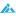# 你真的了解过array_multisort()函数的强大能力吗推荐

01-05 544摘要： 项目中用到这个函数了 ，起初对这个函数一直是懵逼状态，文档都看的朦朦胧胧的。直到今天由于需求的实现详细的了解了这个函数array_multisort。<!----------...

<!----------正文begin-------------->

array_multisort()这个函数可以对多个PHP数组进行排序，排序结果是所有的数组都按第一个数组的顺序进行排列——有点拗口，真的，并且我要只是这样说你一定也不明白。先举个例子：

```<?php
\$a =array(100,80,50,10,0);
\$b = array("c","f","q","e","z");
array_multisort(\$a,\$b);
var_dump(\$a);var_dump(\$b);
?>```

array(5) { => int(0) => int(10) => int(50) => int(80) => int(100) }
array(5) { => string(1) “z” => string(1) “e” => string(1) “q” => string(1) “f” => string(1) “c” }

Warning: array_multisort() [function.array-multisort]: Array sizes are inconsistent in ……

```<?php
//让我们来构造一个多维数组
\$a=array(100,2,4,7,7);
\$ab = array(\$a,\$b);//开始排序
array_multisort(\$ab,SORT_NUMERIC,SORT_DESC,\$ab,SORT_STRING,SORT_ASC);
print_r(\$ab);
?>```

Array (
 => Array (  => 100  => 7  => 7  => 4  => 2 )
 => Array (  => ab  => ag  => ap  => ad  => ac )
)

```<?php
\$array[] = array("age"=>20,"name"=>"li");
\$array[] = array("age"=>21,"name"=>"ai");
\$array[] = array("age"=>20,"name"=>"ci");
\$array[] = array("age"=>22,"name"=>"di");
foreach (\$array as \$key=>\$value){
\$age[\$key] = \$value['age'];
\$name[\$key] = \$value['name'];
}
array_multisort(\$age,SORT_NUMERIC,SORT_DESC,\$name,SORT_STRING,SORT_ASC,\$array);
print_r(\$array);
?>```

Array (
 => Array ( [age] => 22 [name] => di )
 => Array ( [age] => 21 [name] => ai )
 => Array ( [age] => 20 [name] => ci )
 => Array ( [age] => 20 [name] => li )
)

<!----------正文end-------------->

### 觉得文章有用就打赏一下文章作者

#### 支付宝扫一扫打赏#### 微信扫一扫打赏#### 发表评论取消回复01-17 回复